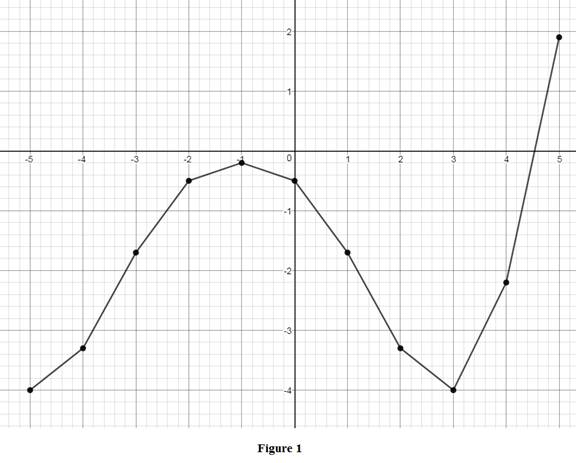# To estimate: The value of f ( g ( x ) ) for x = − 5 , − 4 , − 3 , − 2 , − 1 , 0 , 1 , 2 , 3 , 4 , 5 from the graphs of f and g ; using these values sketch the graph of f ∘ g .### Single Variable Calculus: Concepts...

4th Edition
James Stewart
Publisher: Cengage Learning
ISBN: 9781337687805### Single Variable Calculus: Concepts...

4th Edition
James Stewart
Publisher: Cengage Learning
ISBN: 9781337687805

#### Solutions

Chapter 1.3, Problem 52E
To determine

## To estimate: The value of f(g(x)) for x=−5, −4, −3, −2, −1, 0, 1, 2, 3, 4, 5  from the graphs of f and g; using these values sketch the graph of f∘g.

Expert Solution

### Explanation of Solution

First find the value of g(x).

Note that at x=5, the graph g takes the value of −0.2.

Similarly, from the graph g, the values of g(x) is listed below.

 x g(x) −5 −0.2 −4 1.2 −3 2.2 −2 2.8 −1 3 0 2.8 1 2.2 2 1.2 3 −0.2 4 −1.9 5 −4.1

Table 1

Note that at x=0.2, the graph f takes the value of −4.

Similarly, from the graph f, the values of f(g(x)) is listed below.

 x g(x) f(g(x)) −5 −0.2 −4 −4 1.2 −3.3 −3 2.2 −1.7 −2 2.8 −0.5 −1 3 −0.2 0 2.8 −0.5 1 2.2 −1.7 2 1.2 −3.3 3 −0.2 −4 4 −1.9 −2.2 5 −4.1 1.9

Table 2

Use Table 2 to sketch the graph of fg.

Let x be represented in x-axis and f(g(x)) be represented in y-axis. The graph of fg is shown below in Figure 1.From Figure1, it is observed that the graph of f(g(x)) intersects the x-axis exactly at point.

### Have a homework question?

Subscribe to bartleby learn! Ask subject matter experts 30 homework questions each month. Plus, you’ll have access to millions of step-by-step textbook answers!